## 9.9.2. Subarray Filetype Constructor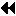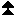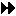Up: Examples Next: Language Bindings Previous: Double Buffering with Split Collective I/O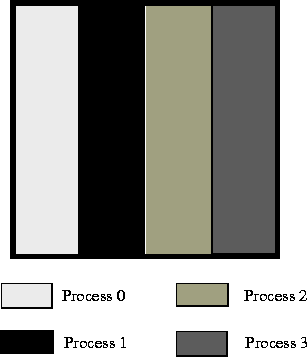Figure 16:

[ ]Example array file layout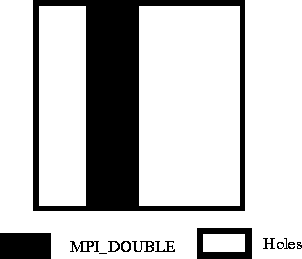Figure 17:

[ ]Example local array filetype for process 1 Assume we are writing out a 100x100 2D array of double precision floating point numbers that is distributed among 4 processes such that each process has a block of 25 columns (e.g., process 0 has columns 0-24, process 1 has columns 25-49, etc.; see Figure 16 ). To create the filetypes for each process one could use the following C program:

```double subarray;
MPI_Datatype filetype;
int sizes, subsizes, starts;
int rank;

MPI_Comm_rank(MPI_COMM_WORLD, &rank);
sizes=100; sizes=100;
subsizes=100; subsizes=25;
starts=0; starts=rank*subsizes;

MPI_Type_create_subarray(2, sizes, subsizes, starts, MPI_ORDER_C,
MPI_DOUBLE, &filetype);
```
Or, equivalently in Fortran:

```double precision subarray(100,25)
integer filetype, rank, ierror
integer sizes(2), subsizes(2), starts(2)

call MPI_COMM_RANK(MPI_COMM_WORLD, rank, ierror)
sizes(1)=100
sizes(2)=100
subsizes(1)=100
subsizes(2)=25
starts(1)=0
starts(2)=rank*subsizes(2)

call MPI_TYPE_CREATE_SUBARRAY(2, sizes, subsizes, starts, &
MPI_ORDER_FORTRAN, MPI_DOUBLE_PRECISION,       &
filetype, ierror)
```
The generated filetype will then describe the portion of the file contained within the process's subarray with holes for the space taken by the other processes. Figure 17 shows the filetype created for process 1.Up: Examples Next: Language Bindings Previous: Double Buffering with Split Collective I/O